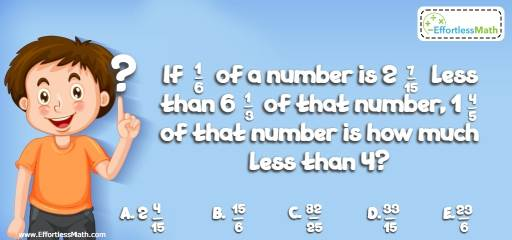# Algebra Puzzle – Challenge 46

This is a perfect mathematics puzzle for those who love to challenge their brains and test their logic skills.## Challenge:

If $$\frac{1}{6}$$ of a number is $$2 \frac{7}{15}$$ less than $$6 \frac{1}{3}$$ of that number, $$1 \frac{4}{5}$$ of that number is how much less than 4?

A- $$2\frac{4}{15}$$

B- $$\frac{15}{6}$$

C- $$\frac{82}{25}$$

D- $$\frac{33}{15}$$

E- $$\frac{23}{6}$$

### The Absolute Best Book to challenge your Smart Student!

Let x be the number. Then:
$$\frac{1}{6 } x = 6 \frac{1}{3 }x – 2 \frac{7}{15} ⇒ 6 \frac{1}{3 }x – \frac{1}{6 } x = 2 \frac{7}{15 } ⇒ \frac{37}{6 }x = \frac{37}{15 }$$
$$X = \frac{2}{5 }$$
Then:
$$1 \frac{4}{5 } x = \frac{9}{5 } × \frac{2}{5 } = \frac{18}{25 }$$
$$4 – (\frac{18}{25 }) = \frac{37}{25 } =\frac{82}{25 }$$

### What people say about "Algebra Puzzle – Challenge 46 - Effortless Math: We Help Students Learn to LOVE Mathematics"?

No one replied yet.

X
30% OFF

Limited time only!

Save Over 30%

SAVE $5 It was$16.99 now it is \$11.99